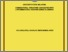Simple Search:

Combinatorial structure associated with low-dimensional filiform leibniz algebras

Citation

Ahmad Jamri, Ayu Ameliatul Shahilah (2017) Combinatorial structure associated with low-dimensional filiform leibniz algebras. Masters thesis, Universiti Putra Malaysia.

Abstract / Synopsis

This thesis is concerned on the studying a graph representation of (n + 1)- dimensional filiform Leibniz algebras. The filiform Leibniz algebras contain three subclasses called first, second and third class that are denoted in dimension n over a field K, by FLbn(K), SLbn(K) and TLbn(K), respectively. This research deals with combinatorial structures associated with FLbn(K) and SLbn(K). Therefore, an algorithm is defined in order to construct such structures associated with filiform Leibniz algebras. By using the table of multiplication of filiform Leibniz algebras, an algorithm for the combinatorial structures associated with filiform Leibniz algebras will be obtained. Next, the structural properties of the combinatorial structure will be constructed to show the non-isomorphism between two classes of filiform Leibniz algebras in such a way of graph theory. Hence, some propositions on combinatorial structures regarding number of vertices and edge, components, degree of vertices, diameter and degree sequences are given. Besides that, an algorithm will be used on association the combinatorial structures with the isomorphism classes of FLbn(K) and SLbn(K). Thus, any two isomorphism classes of FLbn(K) or SLbn(K) are non-isomorphic using combinatorial structures.Preview
PDF
IPM 2018 2 IR.pdfView Item